Journal of Electrical and Electronic Engineering
Volume 4, Issue 5, October 2016, Pages: 109-113

Design and Simulation of PMSM Speed Regulating System Based on SMVSC

Zhou Jianhong1, Ge Debing2, *, Zhang Yulong2

1Electronic & Information Engineering Department, University of Science and Technology Liaoning, Anshan, China

2Applied Technology College, University of Science and Technology Liaoning, Anshan, China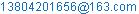(Zhou Jianhong)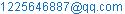(Ge Debing)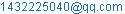(Zhang Yulong)

Zhou Jianhong, GeDebing, Zhang Yulong. Design and Simulation of PMSM Speed Regulating System Based on SMVSC. Journal of Electrical and Electronic Engineering. Vol. 4, No. 5, 2016, pp. 109-113. doi: 10.11648/j.jeee.20160405.14

Received: September 23, 2016; Accepted: October 18, 2016; Published: October 20, 2016

Abstract: Sliding mode variable structure control (SMVSC) has very strong robustness, which has been widely applied in permanent magnet synchronous motor (PMSM) speed regulating system. Constant rate reaching law approach speed is related to the sliding mode reaching gain. The higher the sliding mode reaching gain, the faster the reaching speed, and then the stronger chattering. In this paper, a new reaching law is designed on the basis of constant reaching law. With the analysis of MATLAB, the new reaching law not only increases the approach speed of the sliding mode, but also has a better inhibition effect on chattering. It provides a reference for further experiments.

Keywords: Permanent Magnet Synchronous Motor (PMSM), Sliding Mode Variable Structure Control (SMVSC), Chattering Reduction, MATLAB Simulation

1. Introduction

Permanent magnet synchronous motor(PMSM) has been widely used in the real control engineering for its advantages such as high dynamic performance, high efficiency, simple structure, and small size. So the research on the control technology of PMSM is gradually developed[1-6].The PID controller has such advantages as simple structure and easy realization. It is one of the most common control technologies in the field of PMSM speed regulating system. However, the PID control strategy depends on the model and the parameters of the system. When the system is affected by internal parameters and external disturbance, the conventional PID control can not meet the performance requirements [7-10]. Sliding mode variable structure control (SMVSC) gets people's attention, for its internal parameters perturbation and external disturbance have stronger robustness [11-16]. The constant reaching law has been widely used in the design of SMVSC system for its advantages such as simple structure and easy parameter design [17-18]. For constant reaching law, the greater the gain is, the faster the speed of response is, but the chattering is more obvious. This paper proposes a new reaching law with reduced control chattering, and the response speed improves.

2. PMSM Mathematical Model

The voltage equation: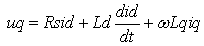(1)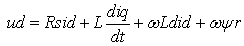(2)

The electromagnetic torque equation: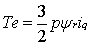(3)

The mechanical operation equation :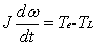(4)

where,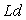,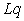is d and q axis inductance of the motor;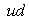,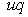is d and q axis voltage of the motor;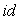,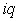d and q axis current of the motor;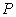is magnetic pole pairs;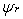is permanent magnet flux linkage;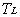is load torque;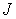is system inertia.

3. The Sliding Mode Variable Structure Control Method

The constant reaching law such as the formula (5):(5)

In this paper, a reaching law is designed on the basis of constant reaching law, as is shown in formula (6):(6)

Where,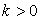,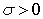,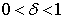. As is known in formula (6) while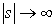, the reaching gain approaching is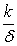. Fromknown,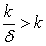, the reaching speed of the sliding mode reaching motion has been improved. While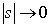, the reaching gain is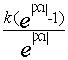. Based on SMVSC,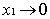, the reaching law gain gradually decreases to zero, and the chattering is reduced.

Selecting the Lyapunov function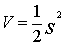to analyze the stability of the formula (6), the stability conditions is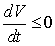. By formula (6) :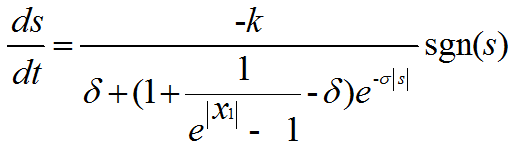(7)

And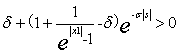, so, the formula (6) satisfies the sliding mode reaching condition.

4. Design of the Controller Based on SMVSC

4.1. Design of the Sliding Mode Surface

The velocity error is defined as: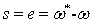(8)

Where,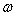*is the given speed,is the actual speed value.(9)

The differential of the sliding mode surface: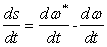(10)

By the mechanical operation equation and the electromagnetic torque equation: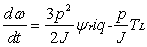(11)

By formula (10) and (11):(12)

By formula (6) and formula (12):(13)

Where,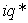is the given by the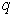axis current controller,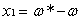is the system state,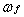is the permanent magnet flux linkage.

4.2. Design of the Load Torque Observer

Formula (13) contains the load torque, but the load torquecan not be measured. Therefore, it is needed to construct an observer to observe the load torque.

The extension state equation of PMSM: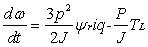(14)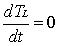(15)

The equation of the sliding mode observer: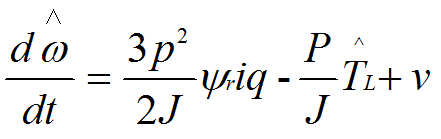(16)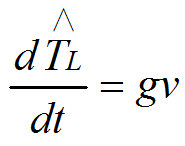(17)

where,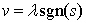,is sliding mode gain, andis the feedback gain.

By formula (14), formula (15), formula (16) and formula (17) to get the observation error equation:(18)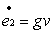(19)

where,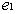is speed observation error, and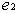is the load torque observation error.

Selecting the sliding mode switching surface, and to analyze the stability by the Lyapunov function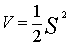, the stability conditions is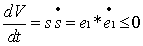. By formula (18),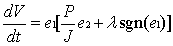. if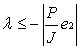, the condition of reach the sliding surface is satisfied. When the system state reaches and remains in the sliding mode surface,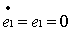. By formula(18) and formula (19):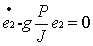(20)

By formula (20), the stability conditions,and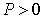,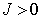,so the range of the feedback gain is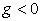. According to the range of the sliding mode gainand the feedback gain, select the reasonable parameters, and the observer can observe the precise load torque.

5. Simulation Analysis

System simulation model is set up in MATLAB/Simulink. The parameter of the constant reaching law is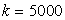.The parameters of the new reaching law are,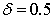,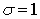. The parameters of PMSM are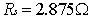,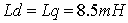,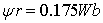,,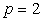,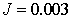. The topology map of the permanent magnet synchronous motor speed regulating system is shown in figure1.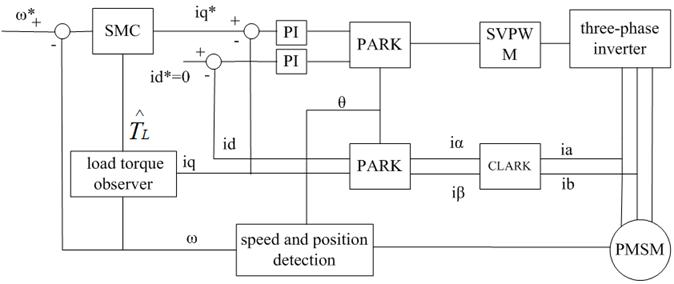Fig. 1. Permanent magnet synchronous motor speed regulating system.

The given speed is 500r/min, and the load torque is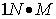. Figure 2 is the motor speed simulation waveforms of the constant reaching law sliding mode control and the new reaching law sliding mode control. Through analysis, they can achieve rapid response speed. For the constant reaching law, the stable time is t=0.15s, but the new reaching law has faster response speed, stable time for t=0.08s. Figure 3is the output waveforms of the current controller under the strategy of the constant reaching law and the new reaching law respectively. The simulation results show that the output fluctuation range of the constant reaching law sliding mode control is -14 to 16A, but for the new reaching law sliding mode control, the output fluctuation range is 6 to 12A. By comparison, it is known that under the new reaching law sliding mode control strategy, the chattering level is less than that under the constant reaching law sliding mode control strategy. That is, the new reaching law sliding mode control has a better inhibitory effect on the chattering.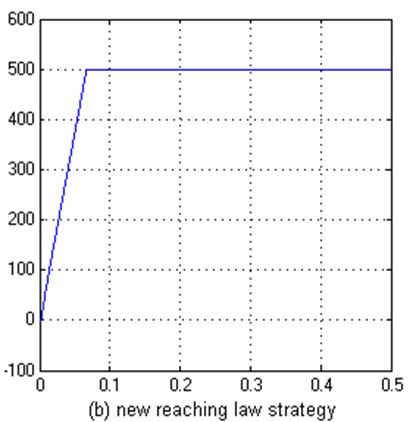Fig. 2. Simulation results of speed.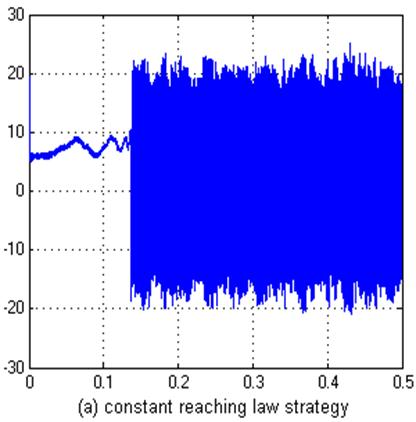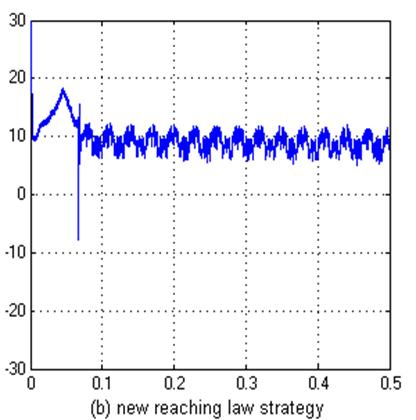Fig. 3. Simulation results of the output wave forms of the current controller.

6. Conclusions

In this paper, the design of a new reaching law is based on constant reaching law, and the simulation analysis of PMSM speed regulating system in MATLAB is carried on. The simulation results show that the new reaching law has a faster dynamic response and also a better inhibition effect on the chattering. The simulation results under the ideal conditions without considering the effects of external disturbance and measurement noise and model uncertainty, etc, so the simulation results can serve as a reference for practical experiments, and the actual effects needs to be verified in further experiments.

References

1. FanweiMeng,Chengying Liu,ZhijunLi,Liping Wang. Adaptive PI control strategy for flat permanent magnet linear synchronous motor vibration suppression[J]. Chinese Journal of Mechanical Engineering,2013,261.
2. GunpyoMaeng,Han Ho Choi. Adaptive sliding mode control of a chaotic nonsmooth-air-gap permanent magnet synchronous motor with uncertainties[J]. Nonlinear Dynamics,2013,743.
3. WahyuKuntoWibowo,Seok-kwon Jeong. Genetic algorithm tuned PI controller on PMSM simplified vector control[J]. Journal of Central South University,2013,2011.
4. Tao Yang,Dale E. Schinstock. Systematic design of current control system for permanent magnet synchronous motors[J]. International Journal of Control, Automation and Systems,2013,116.
5. JiankuiWang,Xiangqing Chen,Jiankuan Fu. Adaptive finite-time control of chaos in permanent magnet synchronous motor with uncertain parameters[J]. Nonlinear Dynamics,2014,782.
6. MihaelaCodrutaAncuti,Lucian Tutelea,Gheorghe-Daniel Andreescu,FredeBlaabjerg,CristianLascu,Ion Boldea. Practical Wide-speed-range Sensorless Control System for Permanent Magnet Reluctance Synchronous Motor Drives via Active Flux Model[J]. Electric Power Components and Systems,2014,421.
7. Li Zheng, Hu Guangda, Cui Jiarui et al.Permanent magnet synchronous motor speed control system of the integral sliding mode variable structure control [J]. Proceedings of the CSEE, 2014,03,pp:431-437.
8. Lai C K,Shyu K K.A novel motor drive design for incremental motion system via sliding-mode control method[J].IEEE Transaction on Industrial Electronics, 2005,52(2):499-507.
9. Zheng Zedong,Li Yongdong,Xiao Xi,et al.Load torque observer of permanent magnet synchronous motor[J].Transactions of China Electrotechnical Society,2010,25(2):30-36.
10. Zhang Xi,Chen Zongxiang,Pan Junmin,et al.Fixed boundary layer sliding mode control of permanent magnet linear synchronous motor[J].Proceedings of the CSEE,2006,26(22):115-121.
11. Roy A M C,Mohammad S I.Application of a sliding mode observer for position and speed estimation in switched reluctance motor drives[J].IEEE Transactions on Industry Application,2001,37(1):51-58.
12. TianYanfeng, GuoQingding. Sliding mode-Hrobustness tracking control for PM linear synchronous motors[J].Transactions of China Electrotechnical Society,2004, 19(4):2-5.
13. Cui Jiarui, Li Qing, Zhang Bo et al. Variable universe adaptive fuzzy PID control of permanent magnet synchronous motor [J]. Proceedings of the CSEE, 2013, S1,PP:190-194.
14. Yi Longlong, Zhang Fuchun, HaoYanzhong. Based on improved sliding mode control of permanent magnet synchronous motor [J].Micro motor, 2013,12,pp:67-70.
15. Wai R.Total sliding-mode controller for PM synchronous servo motor drive using recurrent fuzzy neural network [J].IEEE Transactions on Industrial Electronics,2001,48(5):926-944.
16. Ha Q P,Nllerswithguyen Q H,Rye D C,et al.Fuzzy sliding-mode control applications[J].IEEE Transactions on Industrial Electronics,2001,48(1):38-41.
17. Bin Zhao,Xilin Lu,Minzhe Wu,Zhanxin Mei. Sliding mode control of buildings with base-isolation hybrid protective system[J]. Earthquake Engng. Struct. Dyn.,2000,293.
18. Edwards C., Spurgeon S. K. Sliding mode control:theory and applications [M]. Taylor& Francis, 1998; 28-33.

 Contents 1. 2. 3. 4. 4.1. 4.2. 5. 6.
Article ToolsAbstractPDF(362K)